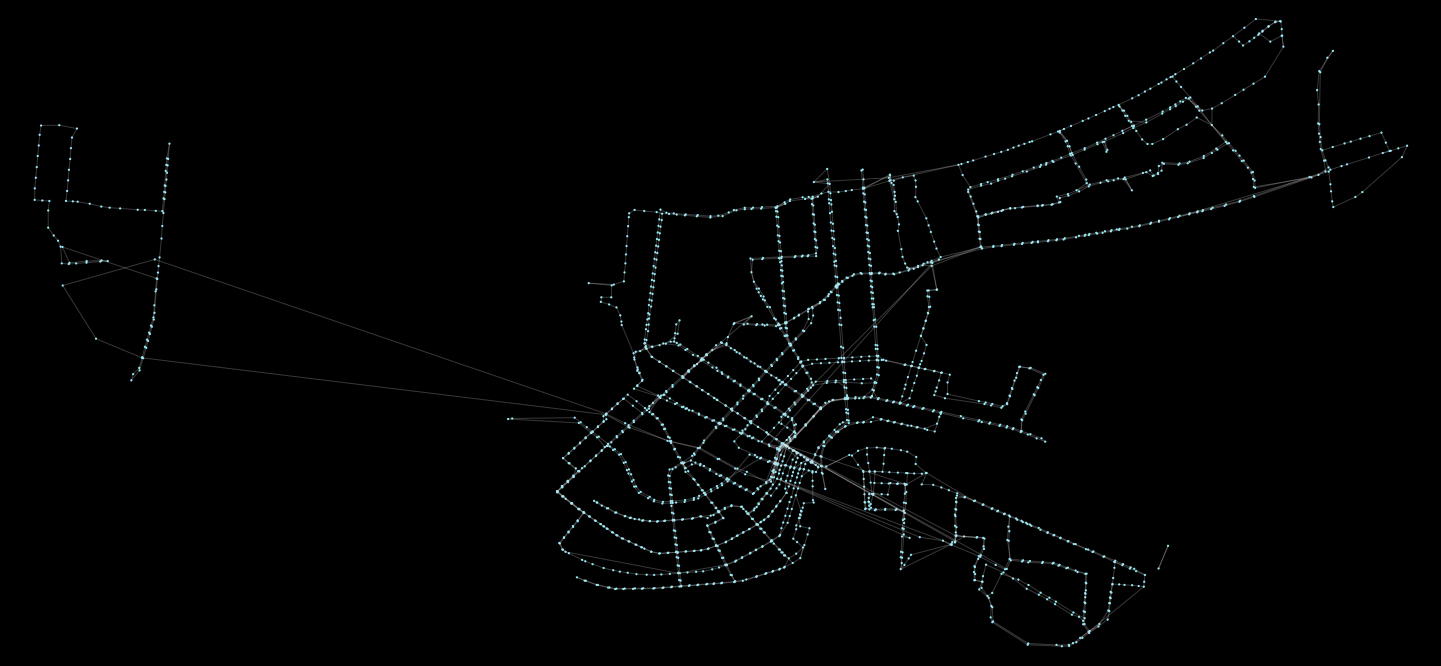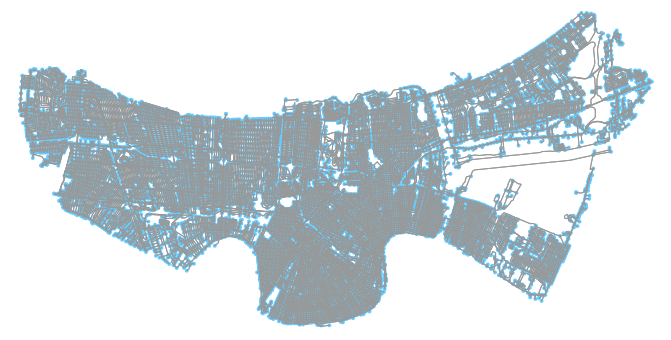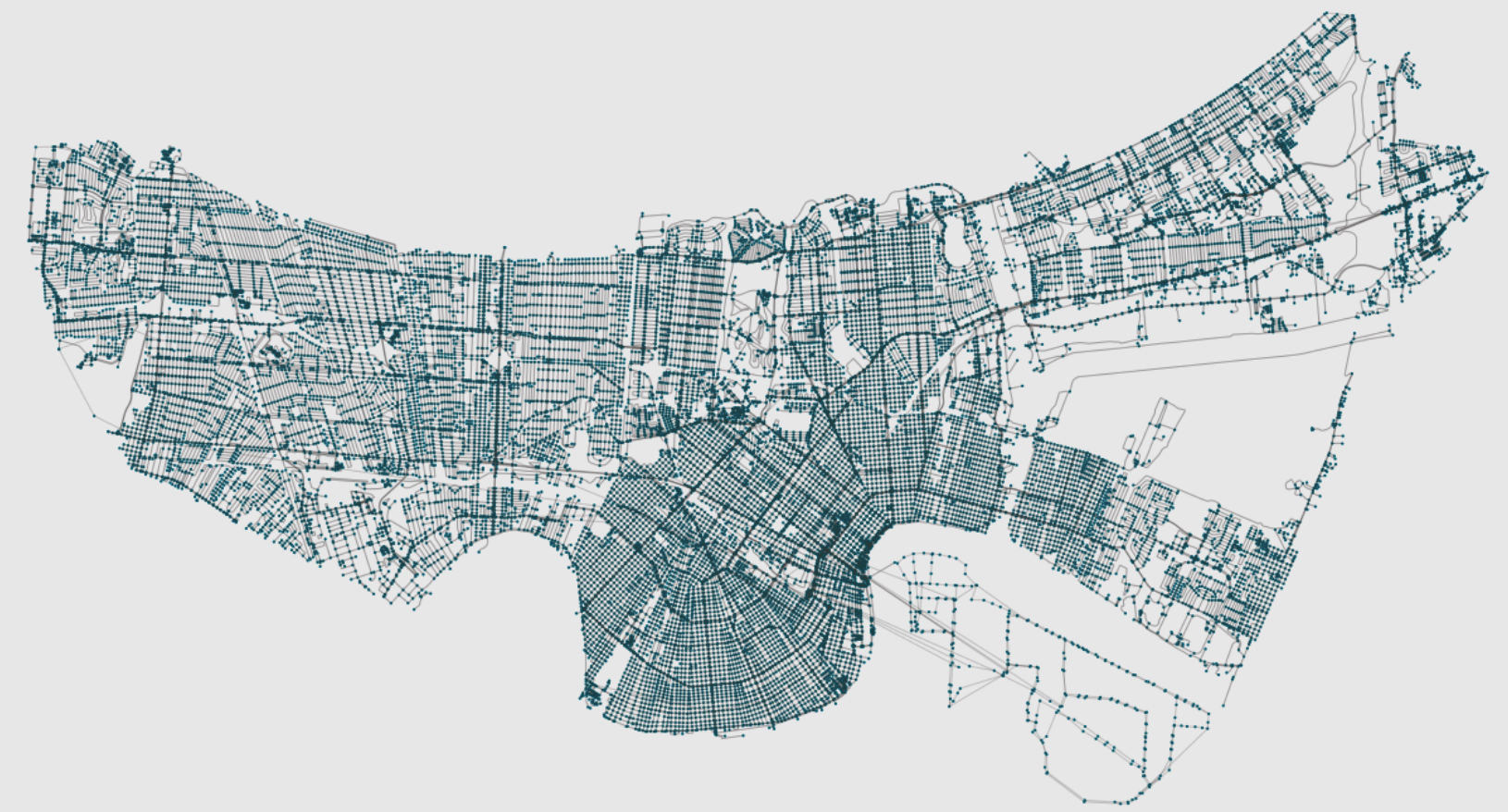## Introduction

Note that the full notebook for this post is held as a Gist on Github, here.

peartree is useful for converting GTFS schedule data into a network graph. It was, in many ways, inspired by OSMnx, which is a tool that converts OpenStreetMap network data to NetworkX graphs. You can read more about OSMnx, here.

This post shows how to use both tools to create a joint network graph that contains both walk (or drive) network data (from OSM, via OSMnx) as well as transit data from a transit operator’s GTFS feed parsed with peartree.

### Dependencies

We will be using peartree version 0.6.1, NetworkX version 2.2, matplotlib version 3.0.2, and OSMnx version 0.8.2.

For the purposes of this example, we will be using the GTFS feed for New Orleans. You can download the latest GTFS feed from New Orleans’ Regional Transit Authority, here.

## Extracting the transit graph

Generate the transit graph as you would any peartree graph.

First, get the representative feed:

Then, pick a target time to extract as a graph (in this case we will do peak hour from seven to nine in the morning.

We can view the results like so:

This will generate the following graphic:## Generating the walk network

The walk network is also as straightforward as using OSMnx’s standard pattern. In this case, though, we first need a boundary. Let’s use the one that is the coverage area of the transit network. We can get that from the graph we just created. Let’s use a few Shapely operations to help accomplish this:

With that result, we can use the new polygon to query OSM for the walk network data to construct a NetworkX graph with:

Once again, let’s plot our new graph. We can use OSM to help us do this:## Merging the two graphs

Now comes the fun part; let’s merge the two graphs together. First let’s observe the structure of the two graphs edges:

Since peartree represents edge length (that is the impedance value associated with the edge) in seconds; we will need to convert the edge values that are in meters into seconds:

We can now check that we do indeed have the original length value preserved:

Now we need to update the nodes. All nodes need to have a boarding cost. We can ensure that all OSMnx nodes have a boarding cost of 0 by simply assigning that value to each of them:

Now that the two graphs have the same internal structures, we can load the walk network onto the transit network with the following peartree helper method:

### Clean up steps

Unfortunately, there’s a few issues that can arise when performing this merge. I intend on eventually working out the kinks - but in the meantime the main thing to check for is hanging nodes. When performing the stacking operation of adding the walk network to the transit network, some nodes can become isolated (attached to nothing else). These isolated subgraphs with no edge count can be removed safely. The following outlines a pattern to use to do so:

## Conclusion

And there you have it - following those simple steps should be sufficient to generate a combined graph. We can now view it as though it were any other peartree graph: